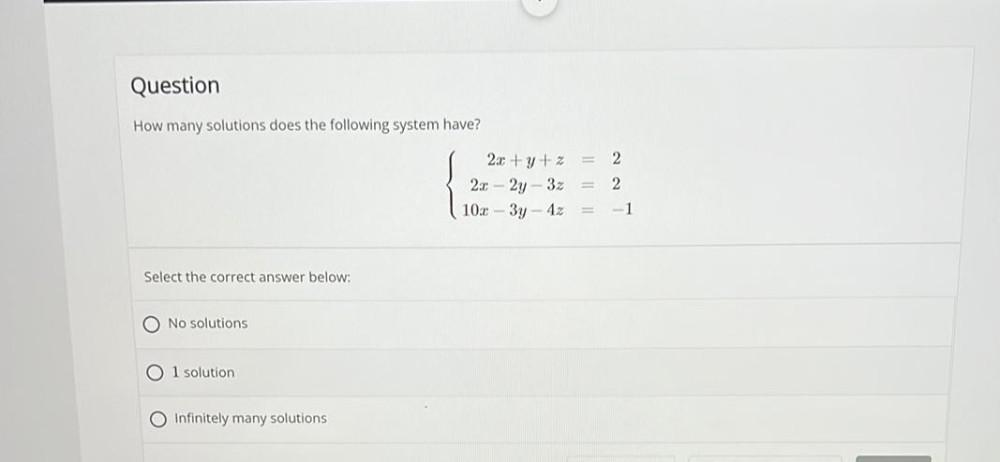Question:

# How many solutions does the following system have? 2x + y + z=2 2x - 2y - 3z=2 10x - 3y - 4z=-1 Select the correct answer below: a) No solutions b)l solution c)Infinitely many solutionsHow many solutions does the following system have? 2x + y + z=2 2x - 2y - 3z=2 10x - 3y - 4z=-1 Select the correct answer below: a) No solutions b)l solution c)Infinitely many solutions